Get instant live expert help with Excel or Google Sheets“My Excelchat expert helped me in less than 20 minutes, saving me what would have been 5 hours of work!”

#### Post your problem and you'll get expert help in seconds

Your message must be at least 40 characters
Our professional experts are available now. Your privacy is guaranteed.

# All values in a range are at least

We can check if all values in a range are greater than or equal to a certain number by independently using or combining the COUNTIF, IF and NOT functions. This tutorial will step through the process.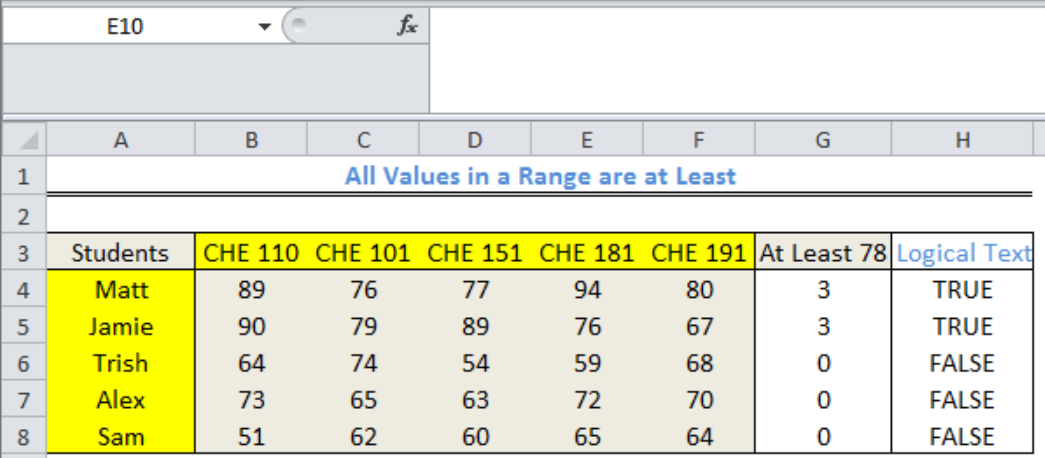Figure 1: All values in a range are at least

## Setting up the Data

We will use data of test scores for 5 students in 5 subjects they offered in a semester.

• We will input the names of the students in Cell A4 to Cell A8
• We will input the test scores in the range B4:F8
• Column G and Column H will contain results that we will get shortly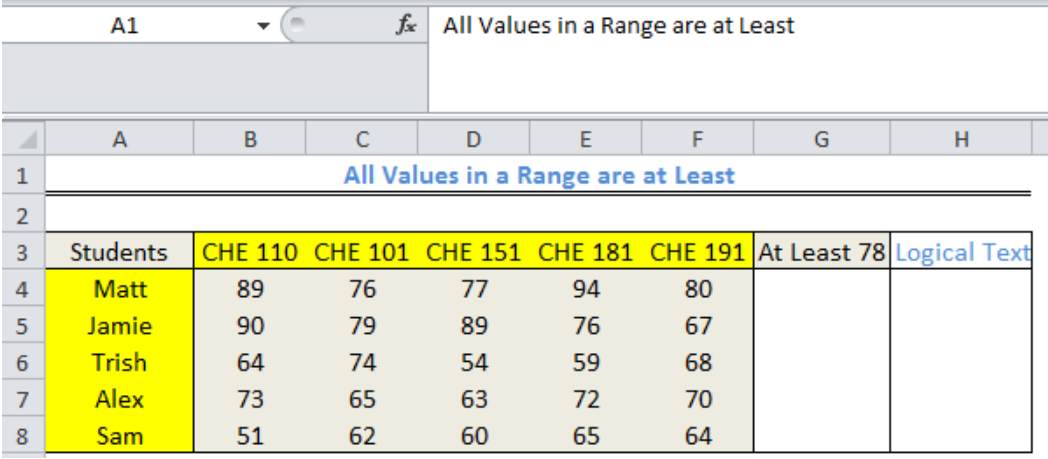Figure 2: Setting up the Data

## Count Subjects where Students Score At Least 78

We will count the number of subjects where each student scored at least 78 by doing the following:

• We will click on Cell G4
• We will input the formula below and press the enter key

`=(COUNTIF(B4:F4,">78"))`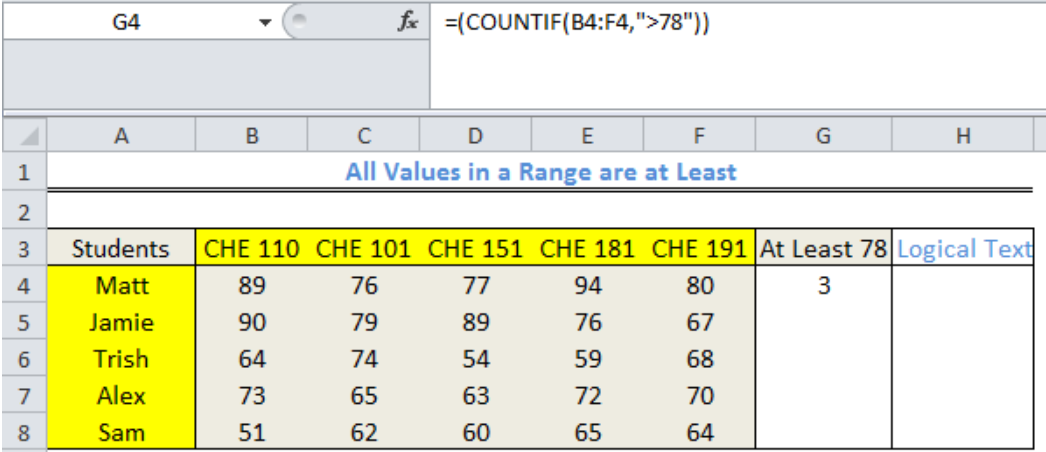Figure 3: Result for Matt showing that he scored At Least 78 in THREE subjects

• We will use the fill handle to copy the formula into the remaining cells of Column G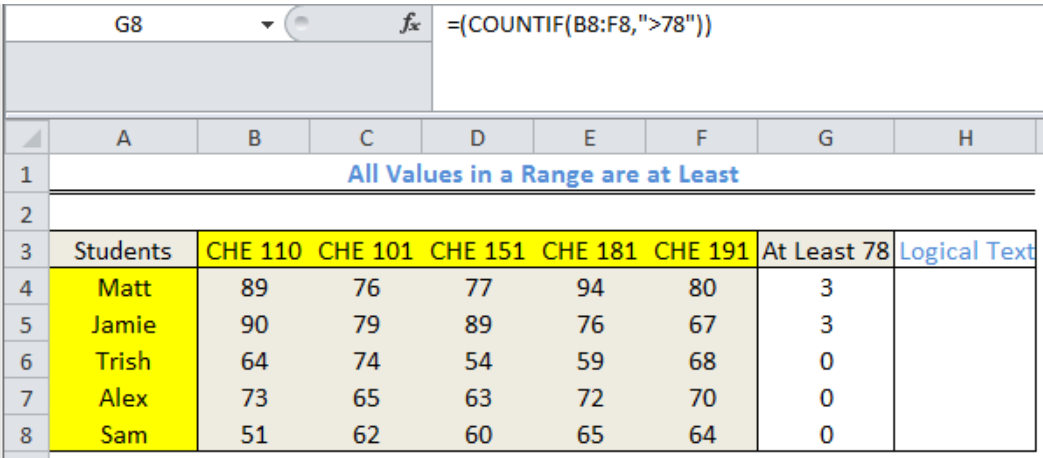Figure 4: Number of subjects where Students scored At Least 78

## Explanation

The COUNTIF function checks the specified range for values that are greater than 78. It returns the count of the number of cells that have values greater than 78 as the result.

## Display TRUE or FALSE if At Least One Value is Less than 78

We can use the IF and COUNTIF functions to display TRUE or FALSE if at least one value meets the criteria within the range

• We will click on Cell H4
• We will input the formula below and press the enter key

`=IF(COUNTIF(B4:F4,">78")TRUE,FALSE)`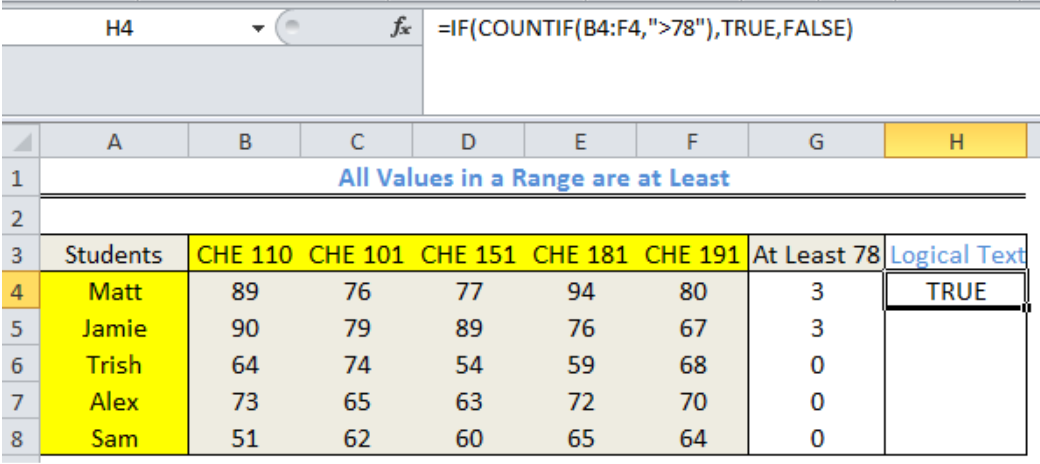Figure 5: Display TRUE or FALSE if At Least One Value is Less than 78

• We will use the fill handle to copy the formula into the remaining cells of Column H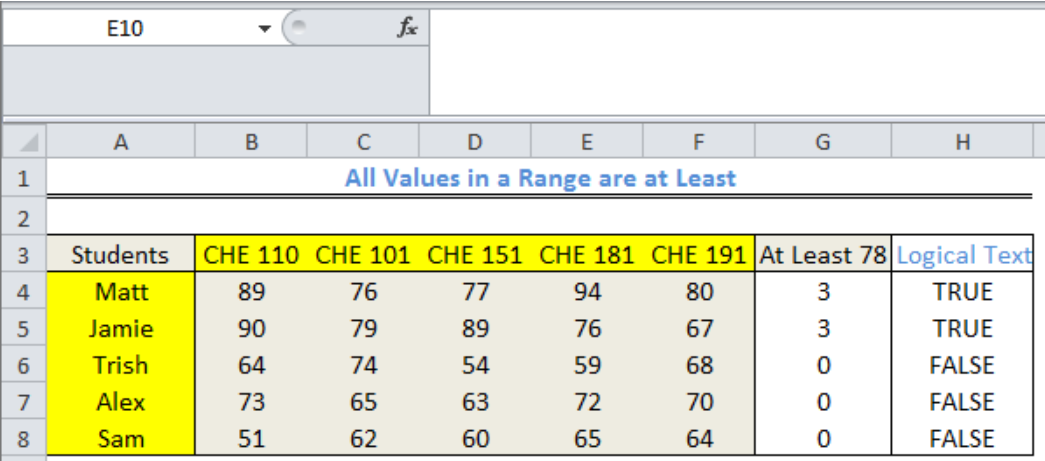Figure 6: Final result displaying TRUE or FALSE if At Least One Value is Less than 78

This result shows that three students scored less than 78 in all subjects.

## Explanation

The COUNTIF function checks the specified range for values that are greater than 78. It returns the count of the number of cells that have values greater than 78. The IF function returns TRUE if the COUNTIF function returns at least a cell that fits the criteria and FALSE if otherwise.

## Note

The NOT function works by returning the opposite of what COUNTIF says. In this formula: `=NOT(COUNTIF(B4:F4,">78"))`, COUNTIF returns three cells with values greater than 78 and the NOT function returns FALSE as the OUTPUT.

## Instant Connection to an Expert through our Excelchat Service

Most of the time, the problem you will need to solve will be more complex than a simple application of a formula or function. If you want to save hours of research and frustration, try our live Excelchat service! Our Excel Experts are available 24/7 to answer any Excel question you may have. We guarantee a connection within 30 seconds and a customized solution within 20 minutes.

### Did this post not answer your question? Get a solution from connecting with the expert.Another blog reader asked this question today on Excelchat:
Related blogs## Subscribe to Excelchat.coAnother blog reader asked this question today on Excelchat: